# Image Super Resolution using ESRGAN

Stay organized with collections Save and categorize content based on your preferences.

This colab demonstrates use of TensorFlow Hub Module for Enhanced Super Resolution Generative Adversarial Network (by Xintao Wang et.al.) [Paper] [Code]

for image enhancing. (Preferrably bicubically downsampled images).

Model trained on DIV2K Dataset (on bicubically downsampled images) on image patches of size 128 x 128.

Preparing Environment

``````import os
import time
from PIL import Image
import numpy as np
import tensorflow as tf
import tensorflow_hub as hub
import matplotlib.pyplot as plt
``````
```2022-12-14 12:38:25.223937: W tensorflow/compiler/xla/stream_executor/platform/default/dso_loader.cc:64] Could not load dynamic library 'libnvinfer.so.7'; dlerror: libnvinfer.so.7: cannot open shared object file: No such file or directory
2022-12-14 12:38:25.224081: W tensorflow/compiler/xla/stream_executor/platform/default/dso_loader.cc:64] Could not load dynamic library 'libnvinfer_plugin.so.7'; dlerror: libnvinfer_plugin.so.7: cannot open shared object file: No such file or directory
2022-12-14 12:38:25.224092: W tensorflow/compiler/tf2tensorrt/utils/py_utils.cc:38] TF-TRT Warning: Cannot dlopen some TensorRT libraries. If you would like to use Nvidia GPU with TensorRT, please make sure the missing libraries mentioned above are installed properly.
```
````wget "https://user-images.githubusercontent.com/12981474/40157448-eff91f06-5953-11e8-9a37-f6b5693fa03f.png" -O original.png`
```
```--2022-12-14 12:38:26--  https://user-images.githubusercontent.com/12981474/40157448-eff91f06-5953-11e8-9a37-f6b5693fa03f.png
Resolving user-images.githubusercontent.com (user-images.githubusercontent.com)... 185.199.108.133, 185.199.109.133, 185.199.110.133, ...
Connecting to user-images.githubusercontent.com (user-images.githubusercontent.com)|185.199.108.133|:443... connected.
HTTP request sent, awaiting response... 200 OK
Length: 34146 (33K) [image/png]
Saving to: ‘original.png’

original.png        100%[===================>]  33.35K  --.-KB/s    in 0.003s

2022-12-14 12:38:26 (11.3 MB/s) - ‘original.png’ saved [34146/34146]
```
``````# Declaring Constants
IMAGE_PATH = "original.png"
SAVED_MODEL_PATH = "https://tfhub.dev/captain-pool/esrgan-tf2/1"
``````

Defining Helper Functions

``````def preprocess_image(image_path):
""" Loads image from path and preprocesses to make it model ready
Args:
image_path: Path to the image file
"""
# If PNG, remove the alpha channel. The model only supports
# images with 3 color channels.
if hr_image.shape[-1] == 4:
hr_image = hr_image[...,:-1]
hr_size = (tf.convert_to_tensor(hr_image.shape[:-1]) // 4) * 4
hr_image = tf.image.crop_to_bounding_box(hr_image, 0, 0, hr_size, hr_size)
hr_image = tf.cast(hr_image, tf.float32)
return tf.expand_dims(hr_image, 0)

def save_image(image, filename):
"""
Saves unscaled Tensor Images.
Args:
image: 3D image tensor. [height, width, channels]
filename: Name of the file to save.
"""
if not isinstance(image, Image.Image):
image = tf.clip_by_value(image, 0, 255)
image = Image.fromarray(tf.cast(image, tf.uint8).numpy())
image.save("%s.jpg" % filename)
print("Saved as %s.jpg" % filename)
``````
``````%matplotlib inline
def plot_image(image, title=""):
"""
Plots images from image tensors.
Args:
image: 3D image tensor. [height, width, channels].
title: Title to display in the plot.
"""
image = np.asarray(image)
image = tf.clip_by_value(image, 0, 255)
image = Image.fromarray(tf.cast(image, tf.uint8).numpy())
plt.imshow(image)
plt.axis("off")
plt.title(title)
``````

#### Performing Super Resolution of images loaded from path

``````hr_image = preprocess_image(IMAGE_PATH)
``````
``````# Plotting Original Resolution image
plot_image(tf.squeeze(hr_image), title="Original Image")
save_image(tf.squeeze(hr_image), filename="Original Image")
``````
```Saved as Original Image.jpg
```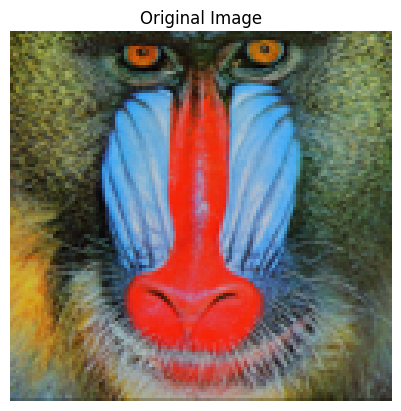``````model = hub.load(SAVED_MODEL_PATH)
``````
```Downloaded https://tfhub.dev/captain-pool/esrgan-tf2/1, Total size: 20.60MB
```
``````start = time.time()
fake_image = model(hr_image)
fake_image = tf.squeeze(fake_image)
print("Time Taken: %f" % (time.time() - start))
``````
```Time Taken: 3.138254
```
``````# Plotting Super Resolution Image
plot_image(tf.squeeze(fake_image), title="Super Resolution")
save_image(tf.squeeze(fake_image), filename="Super Resolution")
``````
```Saved as Super Resolution.jpg
```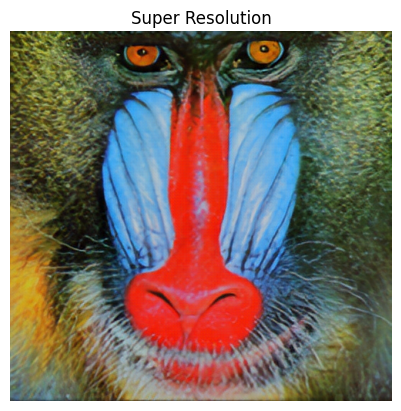### Evaluating Performance of the Model

``````!wget "https://lh4.googleusercontent.com/-Anmw5df4gj0/AAAAAAAAAAI/AAAAAAAAAAc/6HxU8XFLnQE/photo.jpg64" -O test.jpg
IMAGE_PATH = "test.jpg"
``````
```--2022-12-14 12:38:38--  https://lh4.googleusercontent.com/-Anmw5df4gj0/AAAAAAAAAAI/AAAAAAAAAAc/6HxU8XFLnQE/photo.jpg64
HTTP request sent, awaiting response... 200 OK
Length: 84897 (83K) [image/jpeg]
Saving to: ‘test.jpg’

test.jpg            100%[===================>]  82.91K  --.-KB/s    in 0.001s

2022-12-14 12:38:38 (97.1 MB/s) - ‘test.jpg’ saved [84897/84897]
```
``````# Defining helper functions
def downscale_image(image):
"""
Scales down images using bicubic downsampling.
Args:
image: 3D or 4D tensor of preprocessed image
"""
image_size = []
if len(image.shape) == 3:
image_size = [image.shape, image.shape]
else:
raise ValueError("Dimension mismatch. Can work only on single image.")

image = tf.squeeze(
tf.cast(
tf.clip_by_value(image, 0, 255), tf.uint8))

lr_image = np.asarray(
Image.fromarray(image.numpy())
.resize([image_size // 4, image_size // 4],
Image.BICUBIC))

lr_image = tf.expand_dims(lr_image, 0)
lr_image = tf.cast(lr_image, tf.float32)
return lr_image
``````
``````hr_image = preprocess_image(IMAGE_PATH)
``````
``````lr_image = downscale_image(tf.squeeze(hr_image))
``````
```/tmpfs/tmp/ipykernel_45472/2536434270.py:21: DeprecationWarning: BICUBIC is deprecated and will be removed in Pillow 10 (2023-07-01). Use Resampling.BICUBIC instead.
Image.BICUBIC))
```
``````# Plotting Low Resolution Image
plot_image(tf.squeeze(lr_image), title="Low Resolution")
``````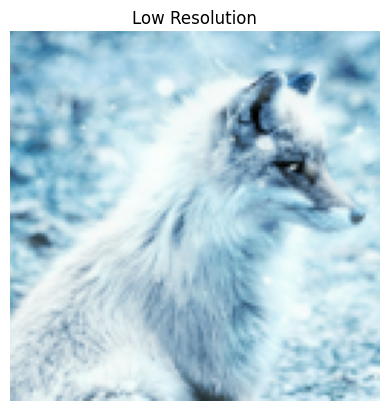``````model = hub.load(SAVED_MODEL_PATH)
``````
``````start = time.time()
fake_image = model(lr_image)
fake_image = tf.squeeze(fake_image)
print("Time Taken: %f" % (time.time() - start))
``````
```Time Taken: 1.121494
```
``````plot_image(tf.squeeze(fake_image), title="Super Resolution")
# Calculating PSNR wrt Original Image
psnr = tf.image.psnr(
tf.clip_by_value(fake_image, 0, 255),
tf.clip_by_value(hr_image, 0, 255), max_val=255)
print("PSNR Achieved: %f" % psnr)
``````
```PSNR Achieved: 28.029171
```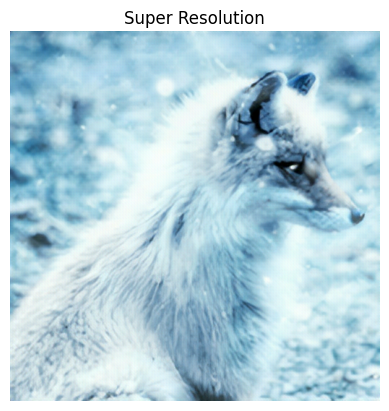Comparing Outputs size by side.

``````plt.rcParams['figure.figsize'] = [15, 10]
fig, axes = plt.subplots(1, 3)
fig.tight_layout()
plt.subplot(131)
plot_image(tf.squeeze(hr_image), title="Original")
plt.subplot(132)
fig.tight_layout()
plot_image(tf.squeeze(lr_image), "x4 Bicubic")
plt.subplot(133)
fig.tight_layout()
plot_image(tf.squeeze(fake_image), "Super Resolution")
plt.savefig("ESRGAN_DIV2K.jpg", bbox_inches="tight")
print("PSNR: %f" % psnr)
``````
```PSNR: 28.029171
```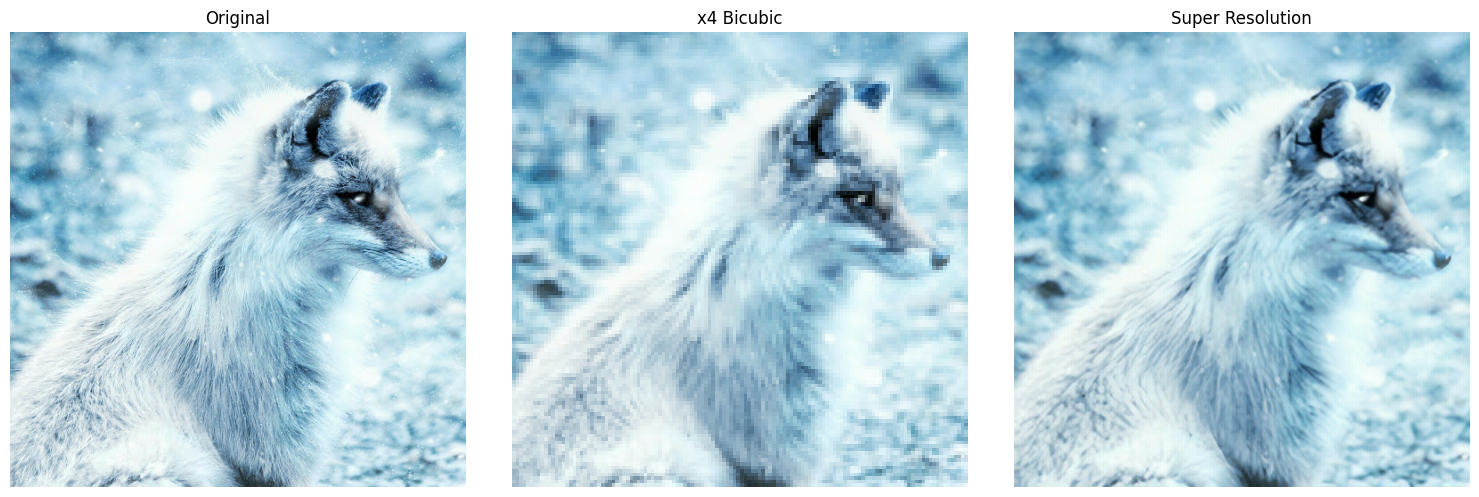[{ "type": "thumb-down", "id": "missingTheInformationINeed", "label":"Missing the information I need" },{ "type": "thumb-down", "id": "tooComplicatedTooManySteps", "label":"Too complicated / too many steps" },{ "type": "thumb-down", "id": "outOfDate", "label":"Out of date" },{ "type": "thumb-down", "id": "samplesCodeIssue", "label":"Samples / code issue" },{ "type": "thumb-down", "id": "otherDown", "label":"Other" }]
[{ "type": "thumb-up", "id": "easyToUnderstand", "label":"Easy to understand" },{ "type": "thumb-up", "id": "solvedMyProblem", "label":"Solved my problem" },{ "type": "thumb-up", "id": "otherUp", "label":"Other" }]